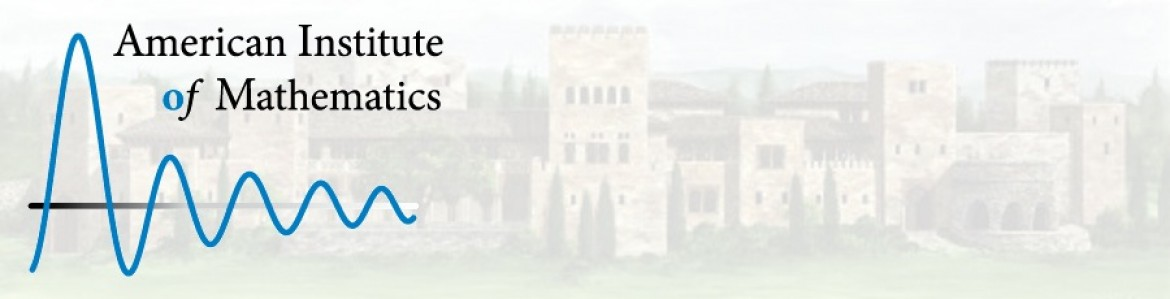Material DetailIntroduction to Probability, Statistics, and Random Processes

This probability and statistics textbook covers: Basic concepts such as random experiments, probability axioms, conditional probability, and counting methods Single and multiple random variables (discrete, continuous, and mixed), as well as moment-generating functions, characteristic functions, random vectors, and inequalities Limit theorems and convergence Introduction to mathematical statistics, in particular, Bayesian and classical statistics Random processes including processing of random signals, Poisson processes, discrete-time and continuous-time Markov chains, and Brownian motion Simulation using MATLAB and R The site includes: The entire textbook Short video lectures that aid in learning the material Online probability calculators for important functions and distributions A... Show More
Rate

Quality

• User Rating
• Learning Exercises
• Bookmark Collections
• Course ePortfolios
• Accessibility Info

Browse...

People who viewed this also viewed
••••Other materials like Introduction to Probability, Statistics, and Random Processes
••••